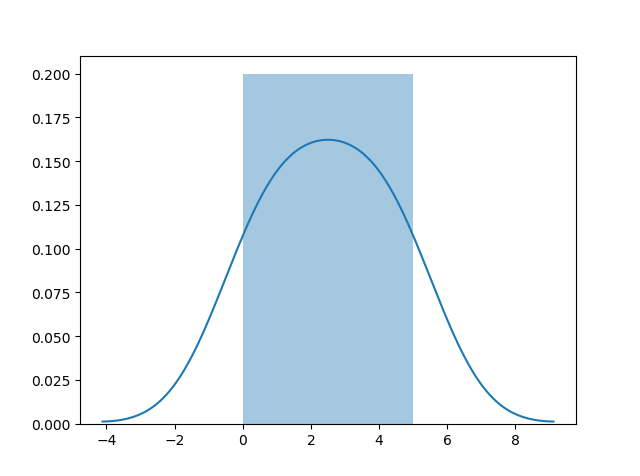THE WORLD'S LARGEST WEB DEVELOPER SITE

# Seaborn

## Visualize Distributions With Seaborn

Seaborn is a library that uses Matplotlib underneath to plot graphs. It will be used to visualize random distributions.## Install Seaborn.

If you have Python and PIP already installed on a system, install it using this command:

If you use Jupyter, install Seaborn using this command:

## Distplots

Distplot stands for distribution plot, it takes as input an array and plots a curve corresponding to the distribution of points in the array.

## Import Matplotlib

Import the pyplot object of the Matplotlib module in your code using the following statement:
import matplotlib.pyplot as plt

You can learn about the Matplotlib module in our Matplotlib Tutorial.

## Import Seaborn

Import the Seaborn module in your code using the following statement:
import seaborn as sns

## Plotting a Displot

### Example

import matplotlib.pyplot as plt
import seaborn as sns

sns.distplot([0, 1, 2, 3, 4, 5])

plt.show()
Try it Yourself »

## Plotting a Distplot Without the Histogram

### Example

import matplotlib.pyplot as plt
import seaborn as sns

sns.distplot([0, 1, 2, 3, 4, 5], hist=False)

plt.show()
Try it Yourself »

Note: We will be using: `sns.distplot(arr, hist=False)` to visualize random distributions in this tutorial.